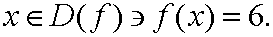MathBits.com presents ...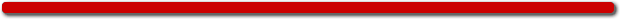.
Welcome to the "PreCalcuCaching"
version of MathCaching!

(This level is for PreCalculus students.)

You are searching for 10 hidden internet boxes. You have just found the first box. Clues to finding the remaining boxes depend upon your ability to solve PreCalculus problems.
Good luck!

Solve the following 3 problems:

1. If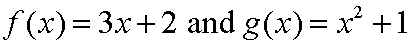,
a) calculate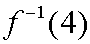b) calculate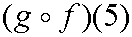c) calculate2. If, which of the number choices represents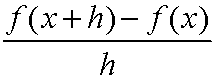:

 1)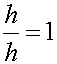2)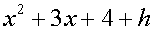3)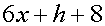4)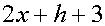3. Given,
find all